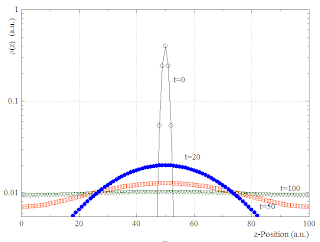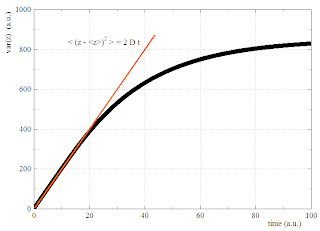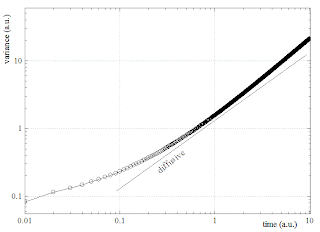## Wednesday, August 12, 2009

###  Cell-Invasion: Implementation of the DDD model

It is possible to solve the Fokker-Planck (FP) equation of post  numerically by direct temporal forward integration, as done before.

Let us discretize space as
z_i = i \; \Delta z

and time as
t_n = n \; \Delta t.

Let \mu be the fraction of density transfered from site i to site i+1 during one time step, so that the corresponding current would be

J_{i,i\!+\!1} = \mu \; \rho_i

and let us denote the density in site i at time n as

\rho^{n}_i = \rho(z_i,t_n).

Except for the most left (i=0) and the most right (i=i_mx) sites (where boundary conditions have to applied properly), the discretized diffusion equation reads

\rho^{n+1}_i  = (1-2\mu) \rho^{n}_i + \mu (\rho^{n}_{i-1} + \rho^{n}_{i+1}).

Note that the physical diffusion constant is related to the discretization parameters as

D = \frac{\mu \Delta z^2}{\Delta t}.

To avoid numerical instabilities, one can set \mu=0.1 and choose a proper time step.

We shall later apply the above method to the DDD model. In this case, \mu is locally replaced by
\mu \rightarrow \mu_i = \mu(\rho^{n}_i).

As a simple test of the method, we start with ordinary diffusion in a box of width 100 with hard walls at z=0 and z=100. The initial density at t=0 is a narrow Gaussian, centered in the middle of the box. The diffusion constant was set to 10.

In a semilog plot, one gets the expected evolution of density:The variance of z-distributions follows initially the behaviour of free diffusion. Later it saturates because of the confinement in the box:Next we turn to the model with density dependent diffusion constant (DDD model). The initial density is now localized in the z=0 layer. The evolution of the particle densities, as resulting from numerical integration of the FP equation, looks as follows:In the semilog. plot one can see the characteristic bi-phasic behaviour and the almost exponential regime at intermediate z.

Here the corresponding cummulative probabilities (= invasion profiles):The variance as a function of time ...... starts sub-diffusively and later appears to become slightly super-diffusive.